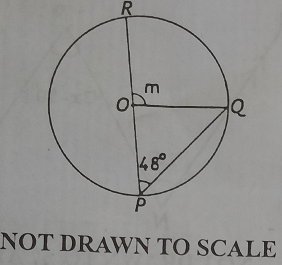mycollegehive
In the diagram, PQR is a circle with center O. If ∠OPQ = 48o, find the value of m
waec-mathematics-2020 math waec-2020 ssce-exam-20200In the diagram, PQR is a circle with center O. If ∠OPQ = 48o, find the value of m

A. 96o

B. 90o

C. 68o

D. 42o

317 viewsShareFollowUniversity of Benin Nigeria
19 August 2020University of Lagos Nigeria
19 August 20200PR is a straight line and the sum of angles on a straight line is 180.

Thus, m + ∠QOP = 180

∠QOP = 180 - m ----------- eqn(1)

Also, |OQP| is a triangle and the sum of all interior angles of a triangle is 180.

Thta is, ∠OPQ + ∠OQP + ∠QOP = 180

∠OPQ = 48

∠OPQ and ∠OQP are in the same segment and angles in a segment are always equal, thus,  ∠OPQ = ∠OQP = 48

Therefore, 48 + 48 + ∠QOP = 180

from eqn(1), ∠QOP = 180 - m

48 + 48 + 180 - m = 180

276 - m = 180

m = 276 - 180

m = 96

Ans AShare### Related Tags

waec-mathematics-2020

15 followers

50 questionsmath

9 followers

1018 questionswaec-2020

8 followers

273 questions